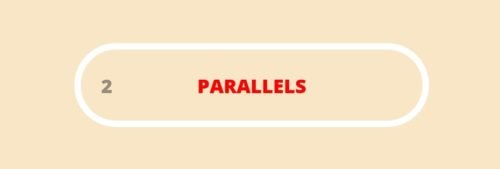# Pythagorea Parallels Level 2.19 Solution/AnswerPythagorea Parallels Level 2.19 New Version Game Answers,  detailed solutions, Tips, and Walkthrough. Scroll below to find answer to this level.

Pythagorea is android/iOS app developed by Horis International Limited. Solutions hints and answers to pythagorea are available in this post scroll down to find solutions to all the levels.

This game is mostly focused on geometric puzzles and construction. The workspace is divided into grids to draw lines. You should know all the basic Math operations. All lines and shapes are drawn on a grid whose cells are squares. Most of the game levels can be answered using natural intuition and by some basic laws of geometry.Pythagorea parallels 2.19: Construct a line through the point A that is parallel to the given line.If you have any doubt regarding Pythagorea answers given here you can watch video below or you can comment on this post-

### 3 thoughts on “Pythagorea Level 2.19 Answer Solution”

•June 16, 2018 at 6:05 am

To Mark: The original line has a horiz run of 5 and a vert rise of 6. The line through A with a 2 unit drop (2 = 6/3) requires a a 5/3 run to the left..

So the answer line must be 1/3 unit off the left wall when it is 2 units below A. To find a point 1/3 unit off the left wall, draw a helper line with a run of 1 and a rise of 6. This helper line will cross at a point of (1/3, 2) which can be used to complete the answer line.

I came here to look up 2-19 answer, I have no connection with either company.

•October 3, 2018 at 9:50 pm
•